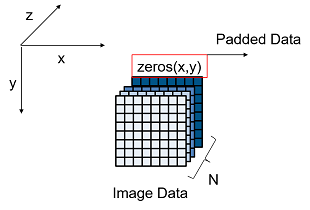Main Content

## External Memory Data Format

To load the input image to the deployed deep learning processor IP core and retrieve the output results, you can read data from the external memory and write data to the external memory by using the `dlhdl.Workflow` workflow. This workflow formats your data. Or, you can manually format your input data. Process the formatted output data by using the external memory data format.

### Key Terminology

• `Parallel Data Transfer Number` refers to the number of pixels that are transferred every clock cycle through the AXI master interface. Use the letter `N` in place of the ```Parallel Data Transfer Number```. Mathematically `N` is the square root of the `ConvThreadNumber`. See ConvThreadNumber.

• `Feature Number` refers to the value of the z dimension of an x-by-y-by-z matrix. For example, most input images are of dimension x-by-y-by-three, with three referring to the red, green, and blue channels of an image. Use the letter `Z` in place of the `Feature Number`.

### Convolution Module External Memory Data Format

The inputs and outputs of the deep learning processor convolution module are typically three-dimensional (3-D).The external memory stores the data in a one-dimensional (1-D) vector. Converting the 3-D input image into 1-D to store in the external memory :

1. Send `N` number of data in the `z` dimension of the matrix.

2. Send the image information along the x dimension of the input image.

3. Send the image information along the y dimension of the input image.

4. After the first `NXY` block is completed, we then send the next `NXY` block along the `z` dimension of the matrix.

The image demonstrates how the data stored in a 3-by-3-by-4 matrix is translated into a 1-by-36 matrix that is then stored in the external memory.When the image `Feature Number` (Z) is not a multiple of the `Parallel Data Transfer Number` (N), then we must pad a zeroes matrix of size x-by-y along the z dimension of the matrix to make the image `Z` value a multiple of `N`.

For example, if your input image is an x-by-y matrix with a `Z` value of three and the value of `N` is four, pad the image with a zeros matrix of size x-by-y to make the input to the external memory an x-by-y-by-4 matrix.

This image is the input image format before padding.This image is the input image format after zero padding.The image shows the example output external memory data format for the input matrix after the zero padding. In the image, A, B, and C are the three features of the input image and G is the zero- padded data to make the input image `Z` value four, which is a multiple of `N`.If your deep learning processor consists of only a convolution (conv) processing module, the output external data is using the conv module external data format, which means it possibly contains padded data if your output `Z` value is not a multiple of the `N` value. The padded data is removed when you use the `dlhdl.Workflow` workflow. If you do not use the `dlhdl.Workflow` workflow and directly read the output from the external memory, remove the padded data.

### Fully Connected Module External Memory Data Format

If your deep learning network consists of both the convolution (conv) and fully connected (fc) layers, the output of the deep learning (DL) processor follows the fc module external memory data format.

The image shows the example external memory output data format for a fully connected output feature size of six. In the image, A, B, C, D, E, and F are the output features of the image.## SupportGet trial now